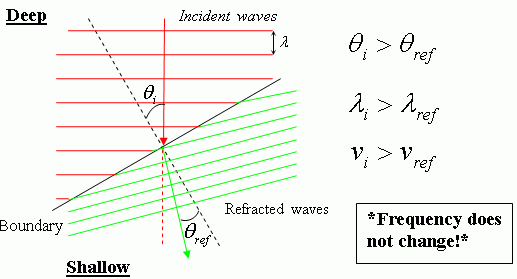#### Reflection of Waves and Pulses

• Transmitted and reflected waves move along the same line of action in a medium but in opposite directions.
• When “positive” water waves hit a straight barrier, they reflect along the same path but as negative waves.
• The speed of the reflected waves is the same as the speed of the transmitted waves
• The wavelength of the reflected waves is the same as the wavelength of the transmitted waves
• Near the barrier destructive interference can be seen as negative pulses meet positive pulses.
• At these points the water seems to be standing still (standing waves are formed here).
• When an incident wave front hits a barrier at an angle other than 900, the reflected waves will bounce back at the same angle to the normal with the barrier.  The angle of incidence is equal to the angle of reflection. (Law of Reflection of waves)
• When straight waves hit a semi-circular barrier they are reflected as circular waves and come together (converge) in one point called the focal point.

#### Refraction of Waves

• If we observe waves traveling from deep water to shallow water in a straight line, we note that while the direction on transmission of the waves does not change both the speed and the wavelength of the wave’s decreases.  The opposite occurs when they travel from shallow to deep waters.
• When waves travel at an angle from deep water to shallow water the direction of motion also changes.
• When waves slow down they bend away from the normal

In general, deep waters are a fast medium for wave travel whereas shallow waters are a slow medium.

Notes from experiments on water waves in a ripple tank (a water tank).

#### Transmission, Reflection, Refraction, and Diffraction

##### Waves in a water tank

In a water tank we observe circular waves as alternate light and dark bands. Waves propagate as circular waves from a single point source “p” in all directions, radially OUTWARD. The distance between successive light bands is one wavelength (λ).

Exampe a stone dropped a point P on the surface of a calm lake or pool will produce circular waves.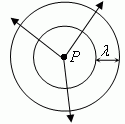##### Reflection of water waves

Circular "incident" waves colliding with a straight barrier are reflected as straight waves.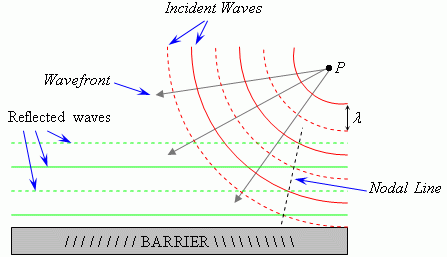The reflected waves interfere with the incident waves and form nodes. Nodes align along nodal lines.

Nodal lines are areas where no wanve energy is transmitted.

Straight waves reflect as straight waves along the same path, parallel to the barrier.

When the barrier is placed at an angle to the source, waves reflect perpendicularly, so that: With respect to a reference line perpendicular to the barrier (this line is called the normal) "the angle of reflection is equal to the incident angle.”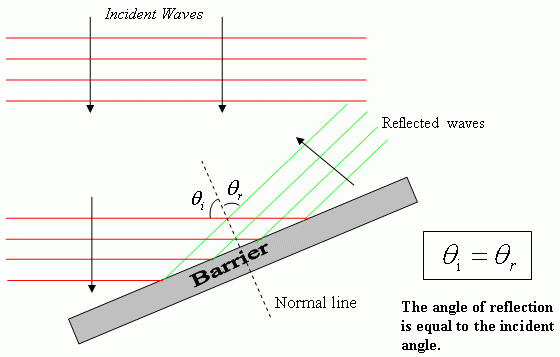Looking at the incident wave fronts in terms of straight lines as individual rays travelling in the same direction of the waves we can illustrate this phenomenon with the following diagram: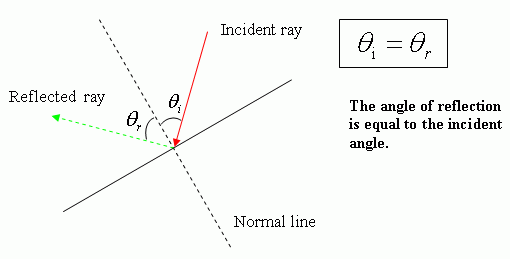Reflection of straight waves from a circular barrier causes the reflected waves to assume a circular pattern: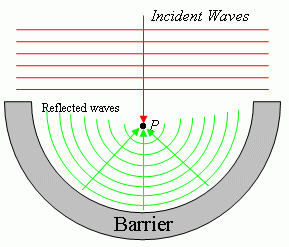##### Refraction of water waves

As straight waves travel from deep water and enter in shallow water their wavelength decreases and their speed also decreases (they slow down). The direction of transmission does not change.

As water waves travel obliquely (at an angle, not straight on) they will change speed and direction according to the following rule:

From deep water to shallow water waves will slow down and bend towards normal.  Therefore we can conclude that since waves bend toward normal, angle of refraction is smaller.